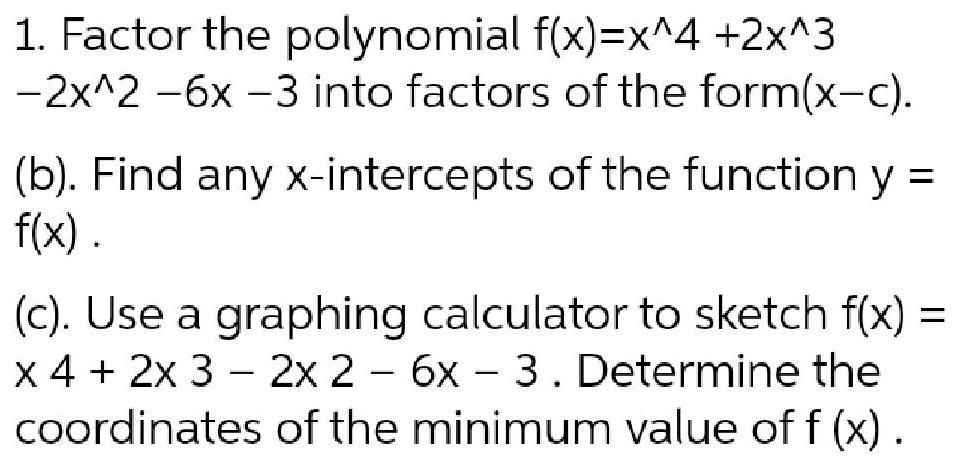Question:

# 1. Factor the polynomial f(x)=x^4 +2x^3-2x^2 -6x -3 into

Last updated: 7/31/20221. Factor the polynomial f(x)=x^4 +2x^3-2x^2 -6x -3 into factors of the form(x-c). (b). Find any x-intercepts of the function y =f(x). (c). Use a graphing calculator to sketch f(x) =x 4 + 2x 3 - 2x 2 - 6x - 3. Determine the coordinates of the minimum value of f(x).